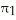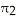/
/
/
1) If we use the χ2 method of analysis to
Not my Question
Flag Content

# Question : 1) If we use the χ2 method of analysis to : 1403637

1) If we use the χ2 method of analysis to test for the differences among 4 proportions, the degrees of freedom are equal to

A) 3.

B) 4.

C) 5.

D) 1.

2) If we wish to determine whether there is evidence that the proportion of items of interest is the same in group 1 as in group 2, the appropriate test to use is

A) the Z test.

B) the χ2 test.

C) Both A and B.

D) Neither A nor B.

3) In testing a hypothesis using the χ2 test, the theoretical frequencies are based on the

A) null hypothesis.

B) alternative hypothesis.

C) normal distribution.

D) None of the above.

TABLE 12-1

A corporation randomly selects 150 salespeople and finds that 66% who have never taken a self-improvement course would like such a course. The firm did a similar study 10 years ago in which 60% of a random sample of 160 salespeople wanted a self-improvement course. The groups are assumed to be independent random samples. Letandrepresent the true proportion of workers who would like to attend a self-improvement course in the recent study and the past study, respectively.

4) Referring to Table 12-1, if the firm wanted to test whether this proportion has changed from the previous study, which represents the relevant hypotheses?

A) H0 : π1 - π2 = 0 versus H1 : π1 - π2 ≠ 0

B) H0 : π1 - π2 ≠ 0 versus H1 : π1 - π2 = 0

C) H0 : π1 - π2 ≤ 0 versus H1 : π1 - π2 > 0

D) H0 : π1 - π2 ≥ 0 versus H1 : π1 - π2 < 0

5) Referring to Table 12-1, what is the critical value when performing a chi-square test on whether the population proportions are different if α = 0.05?

A) ±1.645

B) ±1.96

C) 3.841

D) 2.706

6) Referring to Table 12-1, what is the critical value when testing whether the population proportions are different if α = 0.10?

A) ±1.645

B) ±1.96

C) 3.842

D) 2.706

7) Referring to Table 12-1, what is the value of the test statistic to use in evaluating the alternative hypothesis that there is a difference in the two population proportions using α = 0.10?

A) 4.335

B) 2.706

C) 1.194

D) 0.274

8) Referring to Table 12-1, the company tests to determine at the 0.05 level whether the population proportion has changed from the previous study. Which of the following is most correct?

A) Reject the null hypothesis and conclude that the proportion of employees who are interested in a self-improvement course has changed over the intervening 10 years.

B) Do not reject the null hypothesis and conclude that the proportion of employees who are interested in a self-improvement course has not changed over the intervening 10 years.

C) Reject the null hypothesis and conclude that the proportion of employees who are interested in a self-improvement course has increased over the intervening 10 years.

D) Do not reject the null hypothesis and conclude that the proportion of employees who are interested in a self-improvement course has increased over the intervening 10 years.

9) True or False: In testing the difference between two proportions using the normal distribution, we may use either a one-tail Chi-square test or two-tail Z test.

10) True or False: The squared difference between the observed and theoretical frequencies should be large if there is no significant difference between the proportions.

## Solution 5 (1 Ratings )

Solved
Statistics 1 Year Ago 62 Views